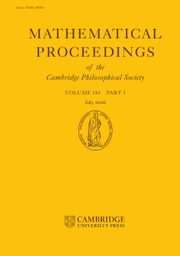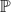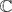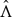Home
Hostname: page-component-78bd46657c-r9wg7 Total loading time: 0.47 Render date: 2021-05-07T12:41:51.714Z Has data issue: true Feature Flags: { "shouldUseShareProductTool": true, "shouldUseHypothesis": true, "isUnsiloEnabled": true, "metricsAbstractViews": false, "figures": false, "newCiteModal": false, "newCitedByModal": true }Mathematical Proceedings of the Cambridge Philosophical Society

# Metric properties of some fractal sets and applications of inverse pressure

Published online by Cambridge University Press:  20 November 2009

Corresponding

## Abstract

We consider iterations of smooth non-invertible maps on manifolds of real dimension 4, which are hyperbolic, conformal on stable manifolds and finite-to-one on basic sets. The dynamics of non-invertible maps can be very different than the one of diffeomorphisms, as was shown for example in [4, 7, 12, 17, 19], etc. In  we introduced a notion of inverse topological pressure P which can be used for estimates of the stable dimension δs(x) (i.e the Hausdorff dimension of the intersection between the local stable manifold Wsr(x) and the basic set Λ, x ∈ Λ). In  it is shown that the usual Bowen equation is not always true in the case of non-invertible maps. By using the notion of inverse pressure P, we showed in  that δs(x) ≤ ts(ϵ), where ts(ϵ) is the unique zero of the function tP(tφs, ϵ), for φs(y):= log|Dfs(y)|, y ∈ Λ and ϵ > 0 small. In this paper we prove that if Λ is not a repellor, then ts(ϵ) < 2 for any ϵ > 0 small enough. In  we showed that a holomorphic s-hyperbolic map on2has a global unstable set with empty interior. Here we show in a more general setting than in , that the Hausdorff dimension of the global unstable set Wu() is strictly less than 4 under some technical derivative condition. In the non-invertible case we may have (infinitely) many unstable manifolds going through a point in Λ, and the number of preimages belonging to Λ may vary. In , Qian and Zhang studied the case of attractors for non-invertible maps and gave a condition for a basic set to be an attractor in terms of the pressure of the unstable potential. In our case the situation is different, since the local unstable manifolds may intersect both inside and outside Λ and they do not form a foliation like the stable manifolds. We prove here that the upper box dimension of Wsr(x) ∩ Λ is less than ts(ϵ) for any point x ∈ Λ. We give then an estimate of the Hausdorff dimension of Wu() by a different technique, using the Holder continuity of the unstable manifolds with respect to their prehistories.

Type
Research Article

## Access options

Get access to the full version of this content by using one of the access options below.

## References

Bedford, E. and Smillie, J.Polynomial diffeomorphisms of2. Invent. Math. 103 (1991), 6999.CrossRefGoogle Scholar
Bowen, R. Equilibrium states and the ergodic theory of Anosov diffeomorphisms. Lecture Notes in Math. 470 (Springer 1973).Google Scholar
Bowen, R.A horseshoe with positive measure. Invent. Math. 29, 203204, 1975.CrossRefGoogle Scholar
Bothe, H. G.Shift spaces and attractors in noninvertible horseshoes. Fund. Math. 152 (1997), no. 3, 267289.Google Scholar
Fornaess, J. E and Sibony, N.Hyperbolic maps on2. Math. Ann. 311 (1998), 305333.CrossRefGoogle Scholar
Katok, A. and Hasselblatt, B.Introduction to the Modern Theory of Dynamical Systems Cambridge University Press (1995).CrossRefGoogle Scholar
Liu, P. D. Invariant measures satisfying an equality relating entropy, folding entropy and negative Lyapunov exponents. Comm. Math. Physics (2008).Google Scholar
Manning, A. and McCluskey, H.Hausdorff dimension for horseshoes. Ergod. Th. and Dyn. Syst. 3 (1983), no. 2, 251260.Google Scholar
Mattila, P.Geometry of Sets and Measures in Euclidian Spaces (Cambridge University Press, 1995).CrossRefGoogle Scholar
Mihailescu, E. Applications of thermodynamic formalism in complex dynamics on2. Discrete and Cont. Dyn. Syst. vol. 7, no. 4 (2001), 821–836.Google Scholar
Mihailescu, E.The set K for hyperbolic non-invertible maps. Ergodic Th. and Dyn. Syst. 22 (2002), 873887.Google Scholar
Mihailescu, E. and Urbanski, M.Estimates for the stable dimension for holomorphic maps. Houston J. Math. 31, no. 2 (2005), 367389.Google Scholar
Mihailescu, E. and Urbanski, M.Inverse topological pressure with applications to holomorphic dynamics of several complex variables. Comm. Contemp. Math. 6, no. 4 (2004), 653682.CrossRefGoogle Scholar
Mihailescu, E. and Urbanski, M. Inverse pressure estimates and the independence of stable dimension. Canad. J. Math. vol. 60, no. 3 (2008), 658–684.Google Scholar
Mihailescu, E. and Urbanski, M. Transversal families of hyperbolic skew products. Discrete and Cont. Dyn. Syst. vol. 21, no. 3 (2008), 907–929.Google Scholar
Nitecki, Z. and Przytycki, F.Preimage entropy for maps. Inter. J. Bifur. Class Appl. Sci. Engr. 9, (1999), no. 9, 18151843.CrossRefGoogle Scholar
Qian, M. and Zhang, Z. S. Ergodic theory for Axiom A endomorphisms. Ergodic Th. and Dyn. Syst. vol. 15, no. 1 (1995), 161–174.Google Scholar
Ruelle, D.Elements of Differentiable Dynamics and Bifurcation Theory (Academic Press, 1989).Google Scholar
Tsuji, M.Fat solenoidal attractors. Nonlinearity 14 (2001), 10111027.CrossRefGoogle Scholar
Walters, P.An Introduction to Ergodic Theory (Springer-Verlag, 1982).CrossRefGoogle Scholar

# Send article to Kindle

Note you can select to send to either the @free.kindle.com or @kindle.com variations. ‘@free.kindle.com’ emails are free but can only be sent to your device when it is connected to wi-fi. ‘@kindle.com’ emails can be delivered even when you are not connected to wi-fi, but note that service fees apply.

Find out more about the Kindle Personal Document Service.

Metric properties of some fractal sets and applications of inverse pressure
Available formats
×

# Send article to Dropbox

To send this article to your Dropbox account, please select one or more formats and confirm that you agree to abide by our usage policies. If this is the first time you use this feature, you will be asked to authorise Cambridge Core to connect with your <service> account. Find out more about sending content to Dropbox.

Metric properties of some fractal sets and applications of inverse pressure
Available formats
×

# Send article to Google Drive

To send this article to your Google Drive account, please select one or more formats and confirm that you agree to abide by our usage policies. If this is the first time you use this feature, you will be asked to authorise Cambridge Core to connect with your <service> account. Find out more about sending content to Google Drive.

Metric properties of some fractal sets and applications of inverse pressure
Available formats
×
×